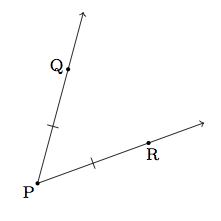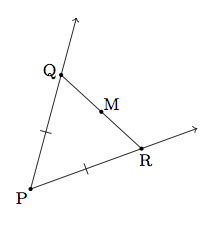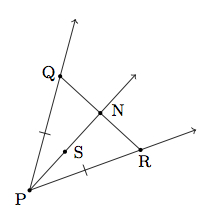# Angle bisection and midpoints of line segments

Alignments to Content Standards: G-CO.D.12

Suppose $P$ is the vertex of an angle and $Q$ and $R$ are points on the two angle rays so that $\overline{PQ}$ and $\overline{PR}$ are congruent:1. If $M$ is the midpoint of $\overline{QR}$ show that $\overrightarrow{PM}$ bisects $\angle QPR$.
2. If ray $\overrightarrow{PS}$ bisects $\angle QPR$ show that $\overrightarrow{PS}$ meets $\overline{QR}$ at its midpoint.

## IM Commentary

This task provides a construction of the angle bisector of an angle by reducing it to the bisection of an angle to finding the midpoint of a line segment. It is worth observing the symmetry -- for both finding midpoints and bisecting angles, the goal is to cut an object into two equal parts. The conclusion of this task is that they are, in a sense, of exactly equivalent difficulty -- bisecting a segment allows us to bisect and angle (part a) and, conversely, bisecting an angle allows us to bisect a segment (part b). In addition to seeing how these two constructions are related, the task also provides an opportunity for students to use two different triangle congruence criteria: SSS and SAS. It is important to note why part (a) is set up to use SSS while part (b) requires SAS. For part (a) we do not know anything about the angles of the triangles but have been given information about all three corresponding side lenghts. For part (b) on the other hand, we have information for two sets of corresponding sides and a one set of corresponding angles.

We note that constructions of this sort come historically from the compass-and-straightedge constructions, but are equally valid in a setting where students use dynamic geometry software to explore geometry. In a software setting students can easily find points $Q$ and $R$ as in the task by constructing a circle centered at $P$. Students could be given the opportunity to use the software to explore the triangles that arise naturally, and then write down conjectures about congruence which they could later be asked to verify. As such, the task is principally intended for instruction or exploration purposes, although as a classic and crucial construction, all students should know this procedure for bisecting an angle. As such, the task is ideal for instruction where the teacher can point out the logical equivalence discussed in the previous paragraph. That said, either part (a) or part (b) in isolation would be suitable for assessment purposes for students being tested on congruence proofs or geometric constructions.

## Solution

1. Pictured below is $\triangle QPR$ with the midpoint $M$ of $\overline{QR}$ added:We can show that $\triangle QPM$ and $\triangle RPM$ are congruent by SSS:

1. Segment $\overline{PQ}$ is congruent to segment $\overline{PR}$ by assumption.
2. Segment $\overline{QM}$ is congruent to segment $\overline{RM}$ because $M$ is the midpoint of segment $\overline{QR}$.
3. Segment $\overline{PM}$ is congruent to segment $\overline{PM}$ since they are the same.

Since $\angle QPM$ and $\angle RPM$ are corresponding angles of congruent triangles, they are congruent and so ray $\overrightarrow{PM}$ bisects angle $A$.

2. Below is a picture of ray $\overrightarrow{PS}$ and the point, labelled $N$, where it meets segment $\overline{QR}$:As in part (a) we will show that $\overrightarrow{PS}$ bisects $\angle QPR$ by showing that $\triangle QPN$ and $\triangle RPN$ are congruent using SAS:

1. Segment $\overline{PQ}$ is congruent to segment $\overline{PR}$ by assumption.
2. Angle $QPN$ and angle $RPN$ are congruent because $\overrightarrow{PN} = \overrightarrow{PS}$ bisects angle $QPR$.
3. Segment $\overline{PN}$ is congruent to segment $\overline{PN}$ since they are the same.

Since $\triangle QPN$ and $\triangle RPN$ are congruent, corresponding sides $\overline{QN}$ and $\overline{RN}$ are congruent which means that $N$ is the midpoint of $\overline{QR}$.